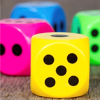Search by Topic

Resources tagged with Comparing and Ordering numbers similar to Dicey Operations:

Filter by: Content type:
Age range:
Challenge level:

There are 15 results

Broad Topics > Numbers and the Number System > Comparing and Ordering numbersDicey Operations

Age 11 to 14 Challenge Level:

Who said that adding, subtracting, multiplying and dividing couldn't be fun?Nice or Nasty

Age 7 to 14 Challenge Level:

There are nasty versions of this dice game but we'll start with the nice ones...Nice or Nasty for Two

Age 7 to 14 Challenge Level:

Some Games That May Be Nice or Nasty for an adult and child. Use your knowledge of place value to beat your opponent.Number Lines in Disguise

Age 7 to 14 Challenge Level:

Some of the numbers have fallen off Becky's number line. Can you figure out what they were?Consecutive Seven

Age 11 to 14 Challenge Level:

Can you arrange these numbers into 7 subsets, each of three numbers, so that when the numbers in each are added together, they make seven consecutive numbers?Mathland Election

Age 11 to 14 Challenge Level:

A political commentator summed up an election result. Given that there were just four candidates and that the figures quoted were exact find the number of votes polled for each candidate.Even Up

Age 11 to 14 Challenge Level:

Consider all of the five digit numbers which we can form using only the digits 2, 4, 6 and 8. If these numbers are arranged in ascending order, what is the 512th number?Snowman

Age 14 to 16 Challenge Level:

All the words in the Snowman language consist of exactly seven letters formed from the letters {s, no, wm, an). How many words are there in the Snowman language?Largest Number

Age 11 to 14 Challenge Level:

What is the largest number you can make using the three digits 2, 3 and 4 in any way you like, using any operations you like? You can only use each digit once.Greetings

Age 11 to 14 Challenge Level:

From a group of any 4 students in a class of 30, each has exchanged Christmas cards with the other three. Show that some students have exchanged cards with all the other students in the class. How. . . .Euromaths

Age 11 to 14 Challenge Level:

How many ways can you write the word EUROMATHS by starting at the top left hand corner and taking the next letter by stepping one step down or one step to the right in a 5x5 array?Writ Large

Age 11 to 14 Challenge Level:

Suppose you had to begin the never ending task of writing out the natural numbers: 1, 2, 3, 4, 5.... and so on. What would be the 1000th digit you would write down.Rachel's Problem

Age 14 to 16 Challenge Level:

Is it true that $99^n$ has 2n digits and $999^n$ has 3n digits? Investigate!Rod Fractions

Age 11 to 14 Challenge Level:

Pick two rods of different colours. Given an unlimited supply of rods of each of the two colours, how can we work out what fraction the shorter rod is of the longer one?Farey Sequences

Age 11 to 14 Challenge Level:

There are lots of ideas to explore in these sequences of ordered fractions.